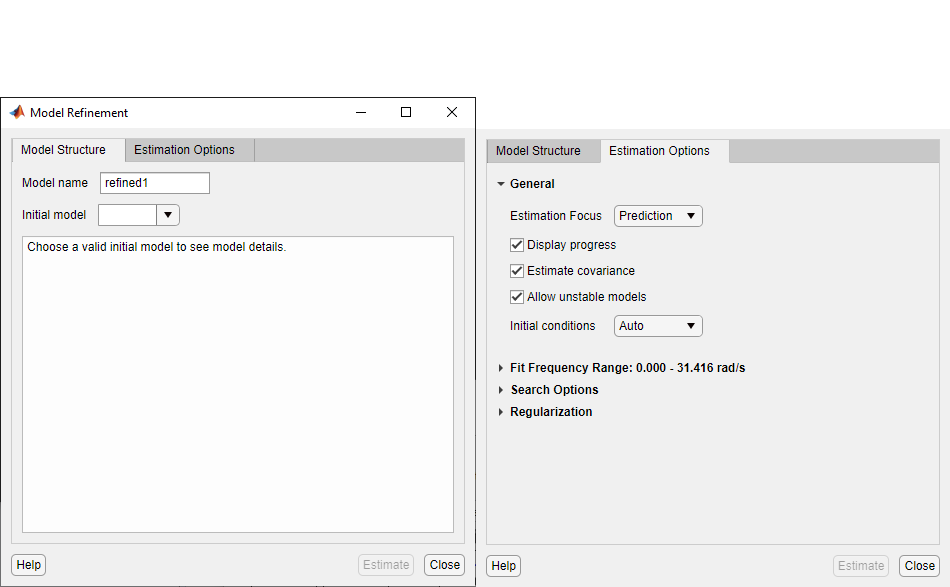Main Content

## Refine Linear Parametric Models

### When to Refine Models

There are two situations where you can refine estimates of linear parametric models.

In the first situation, you have already estimated a parametric model and wish to update the values of its free parameters to improve the fit to the estimation data. This is useful if your previous estimation terminated because of search algorithm constraints such as maximum number of iterations or function evaluations allowed reached. However, if your model captures the essential dynamics, it is usually not necessary to continue improving the fit—especially when the improvement is a fraction of a percent.

In the second situation, you might have constructed a model using one of the model constructors described in Commands for Constructing Linear Model Structures. In this case, you built initial parameter guesses into the model structure and wish to refine these parameter values.

### What You Specify to Refine a Model

When you refine a model, you must provide two inputs:

• Parametric model

• Data — You can either use the same data set for refining the model as the one you originally used to estimate the model, or you can use a different data set.

### Refine Linear Parametric Models Using System Identification App

The following procedure assumes that the model you want to refine is already in the System Identification app. You might have estimated this model in the current session or imported the model from the MATLAB® workspace. For information about importing models into the app, see Importing Models into the App.

To refine your model:

1. In the System Identification app, verify that you have the correct data set in the Working Data area for refining your model.

If you are using a different data set than the one you used to estimate the model, drag the correct data set into the Working Data area. For more information about specifying estimation data, see Specify Estimation and Validation Data in the App.

2. Select Estimate > Refine Existing Models to open the Model Refinement dialog box.For more information on the options in the dialog box, click .

3. Select the model you want to refine in the ```Initial model``` drop-down list or type the model name.

The model name must be in the Model Board of the System Identification app or a variable in the MATLAB workspace. The model can be a state-space, polynomial, process, transfer function or linear grey-box model. The input-output dimensions of the model must match that of the working data.

4. (Optional) Modify the options in the Estimation Options tab.

When you enter the model name, the estimation options in the Model Refinement dialog box override the initial model settings.

• Expand to specify a frequency range over which to fit the data.

• Expand to specify a search method and other search criteria.

• Expand to obtain regularized estimates of model parameters. Specify the regularization constants in the Regularization Options dialog box. To learn more, see Regularized Estimates of Model Parameters.

5. Click to refine the model.

6. Validate the new model. See Ways to Validate Models.

### Refine Linear Parametric Models at the Command Line

If you are working at the command line, you can use `pem` to refine parametric model estimates. You can also use the various model-structure specific estimators — `ssest` for `idss` models, `polyest` for `idpoly` models, `tfest` for `idtf` models, and `greyest` for `idgrey` models.

The general syntax for refining initial models is as follows:

`m = pem(data,init_model)`

`pem` uses the properties of the initial model.

You can also specify the estimation options configuring the objective function and search algorithm settings. For more information, see the reference page of the estimating function.

## SupportGet trial now